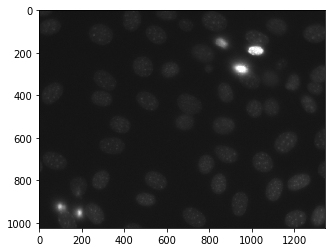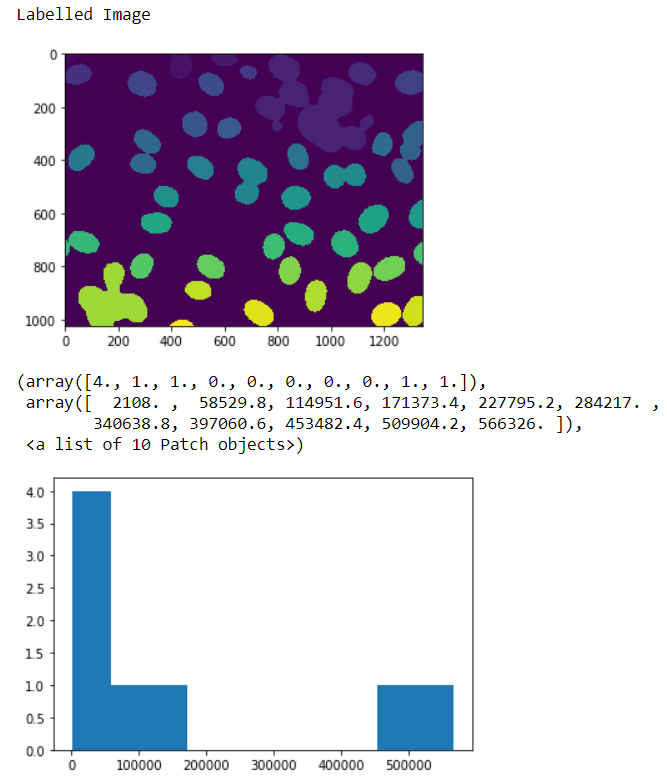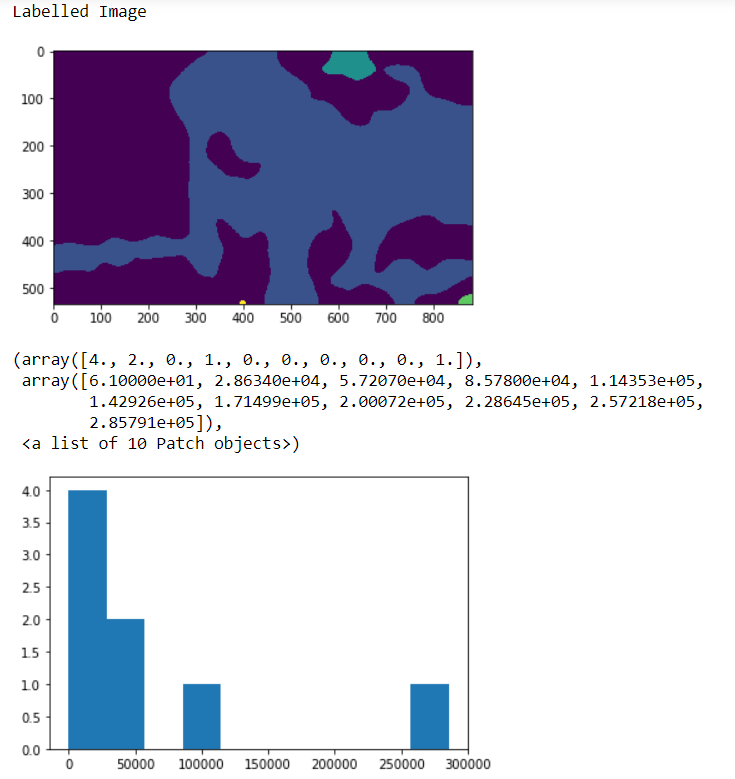Related Articles

# Mahotas – Computing Linear Binary Patterns

• Last Updated : 16 Apr, 2021

In this article we will see how we can get the linear binary patterns of image in mahotas. Local binary patterns is a type of visual descriptor used for classification in computer vision. LBP is the particular case of the Texture Spectrum model proposed in 1990. LBP was first described in 1994. For this we are going to use the fluorescent microscopy image from a nuclear segmentation benchmark. We can get the image with the help of command given below

`mahotas.demos.nuclear_image()`

Below is the nuclear_image

Attention geek! Strengthen your foundations with the Python Programming Foundation Course and learn the basics.

To begin with, your interview preparations Enhance your Data Structures concepts with the Python DS Course. And to begin with your Machine Learning Journey, join the Machine Learning - Basic Level CourseIn order to do this we will use mahotas.features.lbp method

Argument : It takes image object and two integers as argument
Return : It returns 1-D numpy ndarray i.e histogram feature

Note : The input of the this should should be the filtered image or loaded as grey
In order to filter the image we will take the image object which is numpy.ndarray and filter it with the help of indexing, below is the command to do this

`image = image[:, :, 0]`

Example 1 :

## Python3

 `# importing various libraries``import` `mahotas``import` `mahotas.demos``import` `mahotas as mh``import` `numpy as np``from` `pylab ``import` `imshow, show``import` `matplotlib.pyplot as plt` `# loading nuclear image``nuclear ``=` `mahotas.demos.nuclear_image()` `# filtering image``nuclear ``=` `nuclear[:, :, ``0``]` `# adding gaussian filter``nuclear ``=` `mahotas.gaussian_filter(nuclear, ``4``)` `# setting threshold``threshed ``=` `(nuclear > nuclear.mean())` `# making is labelled image``labeled, n ``=` `mahotas.label(threshed)` `# showing image``print``(``"Labelled Image"``)``imshow(labelled)``show()` `# Computing Linear Binary Patterns``value ``=` `mahotas.features.lbp(labelled, ``200``, ``5``)` `# showing histograph``plt.hist(value)`

Output :Example 2 :

## Python3

 `# importing required libraries``import` `numpy as np``import` `mahotas``from` `pylab ``import` `imshow, show``import` `matplotlib.pyplot as plt`` ` `# loading image``img ``=` `mahotas.imread(``'dog_image.png'``)``   ` `# filtering the image``img ``=` `img[:, :, ``0``]``    ` `# setting gaussian filter``gaussian ``=` `mahotas.gaussian_filter(img, ``15``)`` ` `# setting threshold value``gaussian ``=` `(gaussian > gaussian.mean())`` ` `# making is labelled image``labeled, n ``=` `mahotas.label(gaussian)` `# showing image``print``(``"Labelled Image"``)``imshow(labelled)``show()`  `# Computing Linear Binary Patterns``value ``=` `mahotas.features.lbp(labelled, ``200``, ``5``, ignore_zeros ``=` `False``)`  `# showing histograph``plt.hist(value)`

Output :My Personal Notes arrow_drop_up